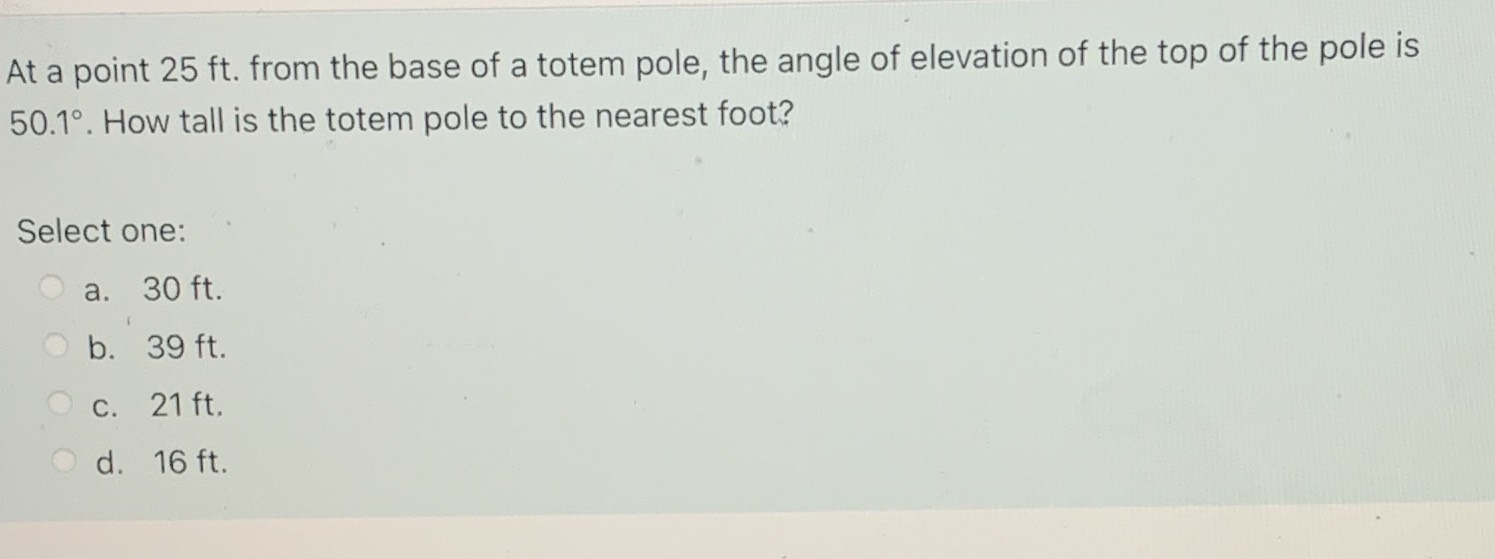### Still have math questions?

Trigonometry
QuestionAt a point $$25 ft$$ . from the base of a totem pole, the angle of elevation of the top of the pole is

$$50.1 ^ { \circ }$$ . How tall is the totem pole to the nearest foot? Select one:

a. $$30 ft$$ . b. $$39 ft$$ . c. $$21 ft$$ . d. $$16 ft$$ .

$$h = 29.89 \approx 30\text{ ft}$$